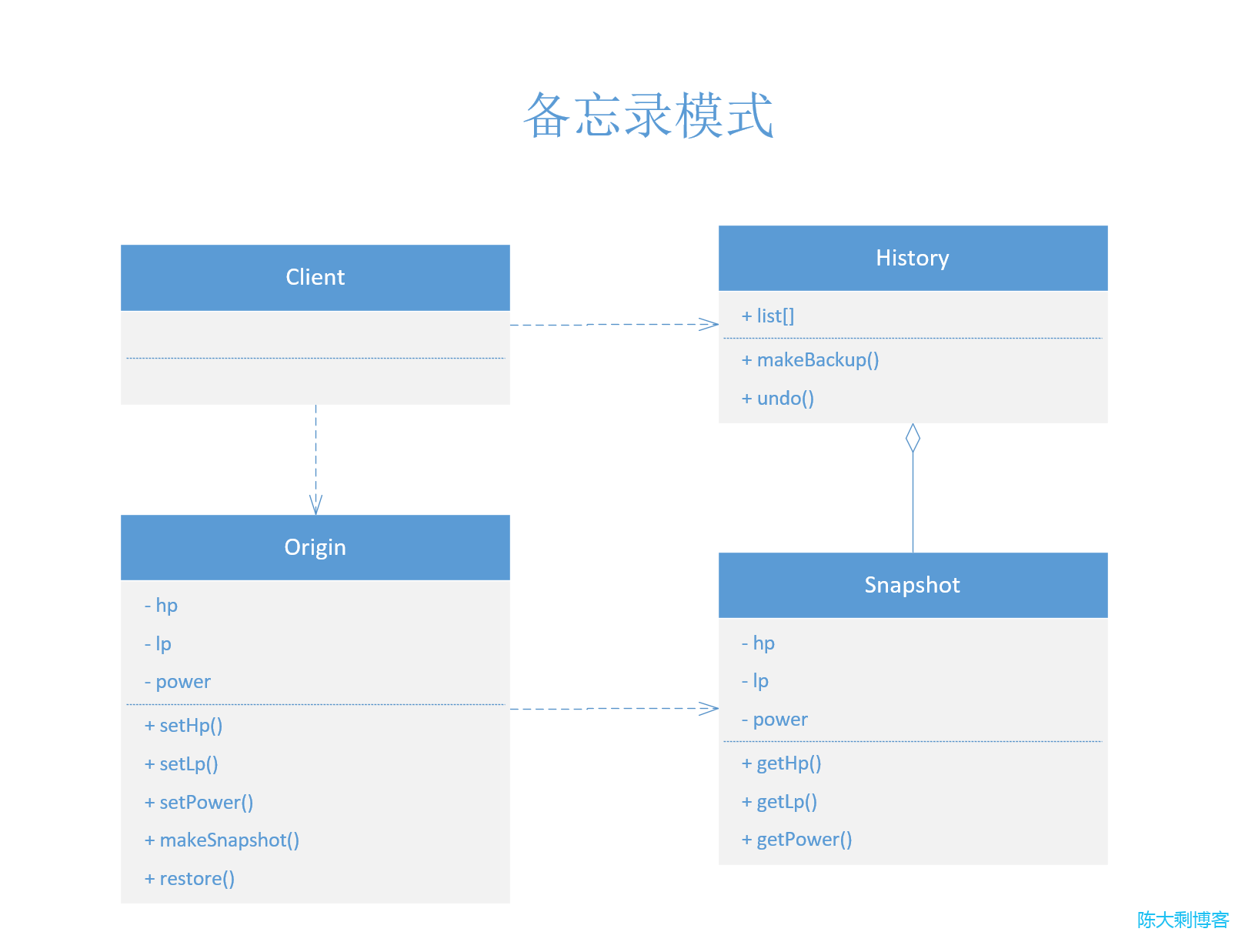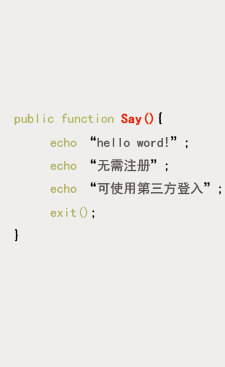# php设计模式(二十一)：备忘录模式（Memento）

• 陈大剩
• 2023-05-23 16:06:39
• 59

## 结构

Origin：原型类 需要创建快照的类
Snapshot：快照类 存原型快照的地方
History：历史类 存储的多个快照 以便能回滚和撤销

## 代码示例

### 原型类

/**
* 原型类
*/
class Origin
{

/**
* 血量值
* @var
*/
private $hp; /** * 体力值 * @var */ private$lp;

/**
* 力量
* @var
*/
private $power; public function __construct() { // 初始化时 HP:100、LP:100、power:50$this->hp = 100;
$this->lp = 100;$this->power = 50;
}

/**
* 设置 HP
* @param $hp * @return void * @author chendashengpc */ public function setHp($hp)
{
$this->hp =$hp;
}

/**
* 设置 LP
* @param $lp * @return void * @author chendashengpc */ public function setLp($lp)
{
$this->lp =$lp;
}

/**
* 设置 Power
* @param $power * @return void * @author chendashengpc */ public function setPower($power)
{
$this->power =$power;
}

/**
* 制作备份
* @return void
* @author chendashengpc
*/
public function makeSnapshot()
{
return new Snapshot($this->hp,$this->lp, $this->power); } /** * 回滚备份 * @return void * @author chendashengpc */ public function restore(Snapshot$snapshot)
{
$this->hp =$snapshot->getHp();
$this->lp =$snapshot->getLp();
$this->power =$snapshot->getPower();
}

/**
* 魔术方法设置字符串
* @return string
* @author chendashengpc
*/
public function __toString()
{
return 'HP：' . $this->hp . PHP_EOL . 'LP：' .$this->lp . PHP_EOL . 'POWERL：' . $this->power . PHP_EOL; } }  ### 快照类 /** * 快照 */ class Snapshot { /** * 血量值 * @var */ private$hp;

/**
* 体力值
* @var
*/
private $lp; /** * 力量 * @var */ private$power;

/**
* 初始化备忘录
* @param $hp * @param$lp
* @param $power */ public function __construct($hp, $lp,$power)
{
$this->hp =$hp;
$this->lp =$lp;
$this->power =$power;
}

/**
* 获取备忘录HP
* @return mixed
* @author chendashengpc
*/
public function getHp()
{
return $this->hp; } /** * 获取备忘录LP * @return mixed * @author chendashengpc */ public function getLp() { return$this->hp;
}
/**
* 获取备忘录POWER
* @return mixed
* @author chendashengpc
*/
public function getPower()
{
return $this->power; } }  ### 历史类 /** * 存历史类 */ class History { public$list = [

];

/**
* 设置快照
* @param Snapshot $snapshot * @return void * @author chendashengpc */ public function makeBackup(Snapshot$snapshot)
{
$this->list[] =$snapshot;
}

/**
* 返回备忘录
* @return mixed|null
* @author chendashengpc
*/
public function undo()
{
if (count($this->list)) {$snapshot = array_pop($this->list); return$snapshot;
}
return null;
}

}


### 客户端使用

/**
* 初始化
*/
$origin = new Origin(); echo$origin . PHP_EOL;
/**
* 提升力量
*/
$origin->setHp(200);$origin->setLp(200);;
$origin->setPower(100); echo$origin . PHP_EOL;

/**
* 存进版本库
*/
$history = new History(); /** * 设置快照 */$history->makeBackup($origin->makeSnapshot());$origin->setHp(120);
$origin->setLp(120);;$origin->setPower(60);
echo $origin . PHP_EOL; /** * 回滚 */$snapshot = $history->undo();$origin->restore($snapshot); echo$origin . PHP_EOL;


HP：100
LP：100
POWERL：50

HP：200
LP：200
POWERL：100

HP：120
LP：120
POWERL：60

HP：200
LP：200
POWERL：100


## UML## 优缺点

### 优点

• 可以在不破坏对象封装情况的前提下创建对象状态快照。
• 可以通过让负责人维护原发器状态历史记录来简化原发器代码。

### 缺点

• 如果客户端过于频繁地创建备忘录， 程序将消耗大量内存。
• 负责人必须完整跟踪原发器的生命周期， 这样才能销毁弃用的备忘录。
• 绝大部分动态编程语言 （例如 PHP、 Python 和 JavaScript）不能确保备忘录中的状态不被修改。

0

## 说点儿什么吧表情

## 留言墙